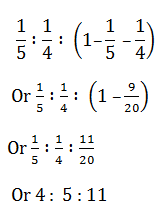# SSC Quantitative Aptitude Questions (Day-64)

Dear Aspirants, you can find the Quantitative Aptitude questions with detailed explanations for the SSC exams. Nowadays the competitive level of the exam has been increasing consistently. Due to the great demand for the government job, the level of the toughness reached greater. Candidates have to enhance the preparation process in order to drive in the right path. It doesn’t need to clear the prescribed cutoff. You must have to score good marks more than the cut off marks to get into the final provisional list. Here we have updating the Quantitative Aptitude questions with detailed explanations on a daily basis. You can practice with us and measure your level of preparation. According to that you can sculpt yourself in a proper way. SSC aspirants kindly make use of it and grab your success in your career.

Start Quiz

1) If N = (√8 + √6)/(√8 – √6), then what is the value of N – (1/N)?

(a) 8√3

(b) 14

(c) 7

(d) 0

2)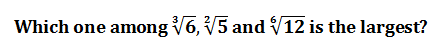(a) ∛6

(b)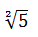(c)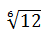(d)  All are equal

3) What is the value of positive square root of 14 + 6√5?

(a) 3 + √5

(b) 3 – √5

(c) 5 + √3

(d) 5 – √3

4)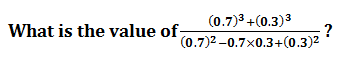(a) 1.2

(b) 0.8

(c) 1

(d) 1.4

5) A boy added all natural numbers from 1 to 10, however he added one number twice due to which the sum becomes 58. What is the number which headded twice?

(a) 3

(b) 4

(c) 7

(d) 8

6) Ram is ﬁve times as efﬁcient as Rohit. Ram can complete a work in 60 days less than Rohit. If both of them work together then in how many days the workwould be completed ?

(a) 33 1/3

(b) 12 1/2

(c) 15

(d) 25

7) A and B together can complete a work in 20 days. They started together but after 4 days A left the work. The remaining work was completed by B alone in24 more days. In how many days can A complete the entire work alone?

(a) 75

(b) 25

(c) 45

(d) 60

8) What will be the net discount (in percentage) after two successive discounts of 20% and 10%?

(a) 28

(b) 30

(c) 32

(d) 42

9) An article is sold for Rs 4080 after a discount of 15%. What is the marked price (in Rs) of the article?

(a) 4600

(b) 4800

(c) 4950

(d) 5150

10) A, B and C are partners and invest in a business. A invests 1/5th of total and B invests 1/4th of the total. What is the ratio of proﬁt of A, B and Crespectively?

(a) 5 : 4 : 9

(b) 5 : 4 : 1

(c) 4 : 5 : 1

(d) 4 : 5 : 11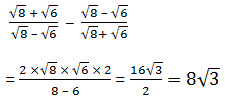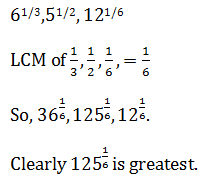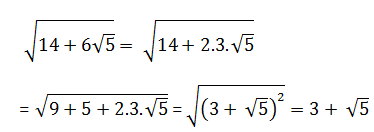0.7+0.3=1

1 + ……. + 10 + x = 58

5 × 11 + x = 58

⟹ x = 3

Ram = 5x Rohit

Rohit × x = Ram × x – 60

Or Rohit × x = 5x Rohit (x – 60)

Or x = 5x – 300

Or 4x = 300

Or x = 75

∴ Rohit completes work in 75 days

∴ Ram completes work in 15 days

So, together = (75×15)/90 = 5/6 × 15

= 5/2 × 5 = 121/2 Days

clearly,

4/20 + 24/B = 1

Or B = 30 days

∴ A = (30 × 20)/ (30 – 20) = 60 days

Net discount % = 20 + 10 – 2 = 28%

4080 = 0.85 MP

(4080 × 100)/85 = MP

Or 4800 = MP